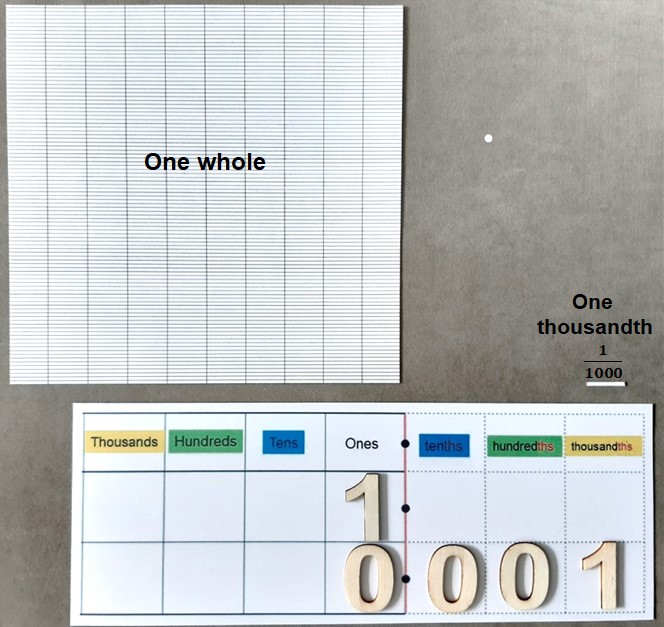## Decimal place value

Using a place value grid, we can see that as digits move to the left, their value is being multiplied by 10 and becomes 10 times larger. As they move to the right, their value is being divided by 10 and becomes 10 times less.If we move a digit in the 'ones' column, one place to the right, we are dividing it by 10 and it moves out of the whole number part of the place value grid and into the decimal part, ending in the first decimal place. A decimal point acts as a barrier between the whole number and the decimal parts of the grid. If there are no whole ones, we usually record a zero in the ones place.

When we take a whole one and divide it up into 10 equal pieces, each fractional piece would be 1/10 so the value of digits in the first decimal place is tenths.When we take a whole one and divide it up into 100 equal pieces, each fractional piece would be 1/100 so the value of digits in the second decimal place is hundredths.When we take a whole one and divide it up into 1000 equal pieces, each fractional piece would be 1/1000 so the value of digits in the second decimal place is thousandths.If we put a line on the ones column, we can see that there is symmetry in the place value on either side. The ‘ths’ on the end of the decimal values is important as it indicates that the value is that sized fraction of a whole one.